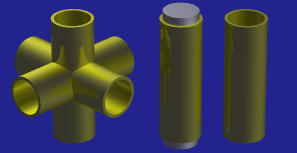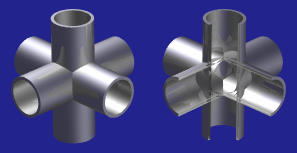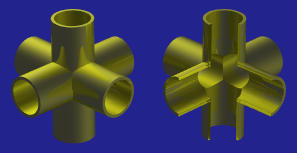# A CSG Design ExampleLet us deign three perpendicular tubes as shown below left.An immediate reaction is that let us subtract a smaller cylinder from a larger one, yielding a tube which is shown in below right. Then, take two more instances of this tube, each of which is rotated an appropriate angle; compute the union of these three tubes; and the result is shown in the figure below.But, the result is not quite right, because the inner junction of the tubes are blocked as seen in the right figure above. A correct solution is to design two instances of three perpendicular cylinders, one larger and the other smaller. Then, subtracting the smaller from the larger yields the designed result as shown below.